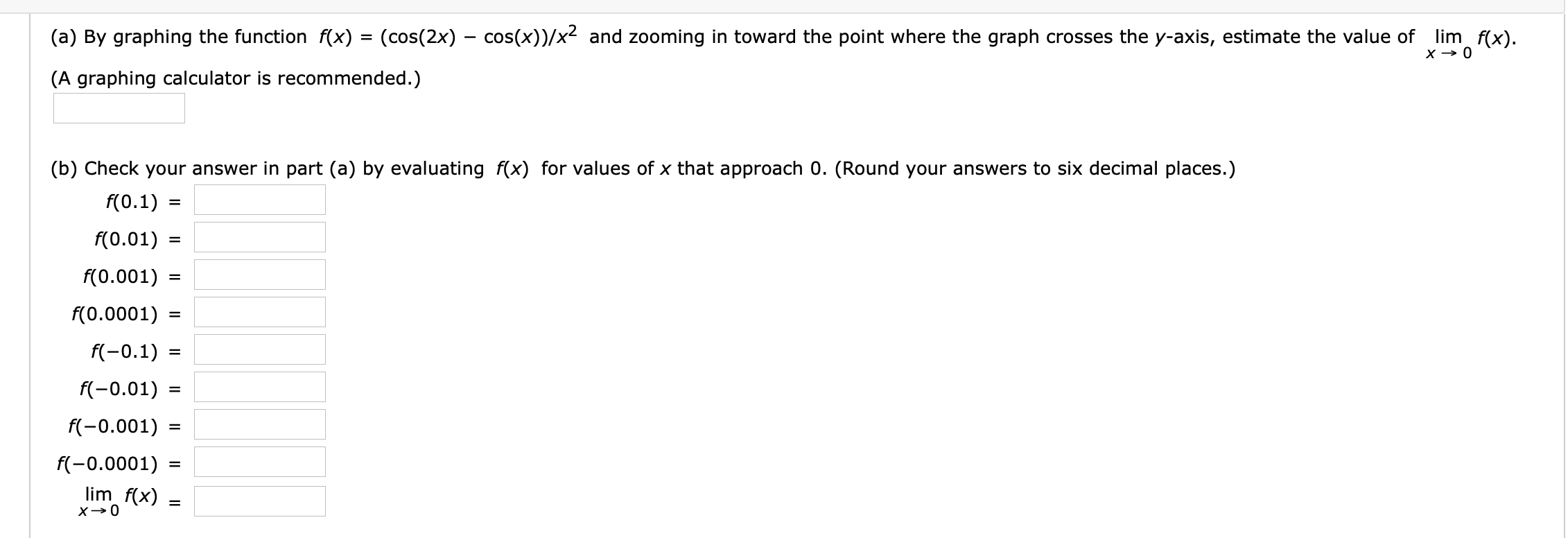(a) By graphing the function f(x) = (cos(2x) - cos(x))/x and zooming in toward the point where the graph crosses the y-axis, estimate the value of lim f(x).(A graphing calculator is recommended.)(b) Check your answer in part (a) by evaluating f(x) for values of x that approach 0. (Round your answers to six decimal places.)f(0.1)f(0.01)f(0.001)=f(0.0001)f(-0.1)=f(-0.01)=f(-0.001)=f(-0.0001)lim f(x)x0

Questionhelp_outlineImage Transcriptionclose(a) By graphing the function f(x) = (cos(2x) - cos(x))/x and zooming in toward the point where the graph crosses the y-axis, estimate the value of lim f(x). (A graphing calculator is recommended.) (b) Check your answer in part (a) by evaluating f(x) for values of x that approach 0. (Round your answers to six decimal places.) f(0.1) f(0.01) f(0.001) = f(0.0001) f(-0.1) = f(-0.01) = f(-0.001) = f(-0.0001) lim f(x) x0 fullscreen
Step 1

Graphed the function using graphing calculator,

zooming in got lim x->0 f(x)= -1.5

Step 2

"Using calculator fou...

Want to see the full answer?

See Solution

Want to see this answer and more?

Our solutions are written by experts, many with advanced degrees, and available 24/7

See Solution
Tagged in

Limits